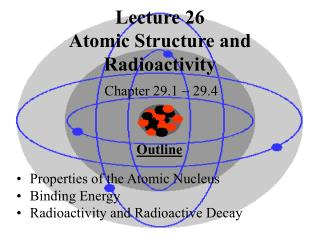DownloadDownload PresentationLecture 26 Atomic Structure and Radioactivity

# Lecture 26 Atomic Structure and Radioactivity

Télécharger la présentation## Lecture 26 Atomic Structure and Radioactivity

- - - - - - - - - - - - - - - - - - - - - - - - - - - E N D - - - - - - - - - - - - - - - - - - - - - - - - - - -
##### Presentation Transcript

1. Lecture 26Atomic Structure and Radioactivity Chapter 29.1  29.4 Outline • Properties of the Atomic Nucleus • Binding Energy • Radioactivity and Radioactive Decay

2. The nucleus contains protons and neutrons, collectively called nucleons. Nuclear Structure Atoms of different chemical elements have different number of protons (atomic number) Atoms with the same number of protons and different number of neutrons are called isotopes A nucleus with a particular composition is called a nuclide. 11H – hydrogen atom with 1 proton in the nucleus

3. Atomic Structure

4. Size of the Nucleus The nucleus size was found by E. Rutherford in particle scattering experiments. In head-on collisions, the entire kinetic energy is converted to electrical potential energy at the particle stopping point. particle charge 1 q1q2 (2e) (Ze) mv2 = ke = ke  2 r r Target nucleus: charge size 4keZe2 r =  mv2 A  number of nucleons r0 = 1.2 1015 cm r = r0 A1/3

5. Radioactivity Radioactivity was discovered accidentally in 1896. Henri Becquerel found that uranium (U) exposed a photographic plate. In other words, uranium emitted penetrating radiation. Marie and Pierre Curie soon discovered 2 more radioactive elements, called polonium and radium. Radioactivity is associated with the nucleus and is not affected by chemical reactions or heating.

6. Radioactive Decay It was found that magnetic field splits the radiation from radioactive materials into 3 parts. Alpha () particles  positively charged Beta () particles  negatively charged  electrons Gamma () particles (rays)  no charge - decay: the nucleus composition does not change. -decay occurs in large, unstable, nuclei. The heaviest stable isotope is 20983Bi. -decay transforms a neutron into a proton (n  p+ + e) Electron capture: p+ + e  n and p+  n + e+

7. Half-Life The half-life of a radionuclide is the period of time needed for half of any its initial amount to decay. 1 mg 22688Ra  0.5 mg 22286Rn for 1600 years Radionuclides have unchanging half-life. This is the base for archaeological dating. Half-life for 14C is 5730 years. Radiation from radionuclides carries high energy. It is harmful for living tissues. 1 sievert (Sv)  1 J of radiation from X-rays or -rays absorbed by 1 kg of body tissue. A permissible limit is ~ 20mSv a year.

8. Half-Life

9. The Decay Constant N  number of radionuclides at some moment of time t N  number of nuclei that decay in a time interval t   decay constant N0  initial number of nuclei T1/2  half-life e = 2.718 N/t  N(t) N = Nt  N(t) = N0 e t N0/2 = N0 e T1/2  T1/2 = 0.693/

10. Nuclear Energy Our everyday life units for energy and mass are not suitable for atoms. The atomic mass unit (unified mass unit): 1u = 1.66 1027 kg Mass of a hydrogen atom is 1.0078 u The energy unit is the electronvolt (eV). 1eV = 1.60 1019 J 1Mev = 1.60 1013 J E (1 u) = mc2 = 931 MeV

11. Binding Energy Hydrogen has 3 isotopes: protium, deuterium, and tritium. The mass of 11H is 1.0078 u , the mass of a neutron is 1.0087 u . The sum is 2.0165 u . The mass of a deuterium atom is 2.0141 u: 0.0024 u = 2.2 MeV less than the combined mass of p+n. All atoms have smaller masses than the sum of masses of their nucleons. Some mass and energy is given off due to action of forces that hold the protons and neutrons together. This energy is called binding energy.

12. Binding Energy Binding energy of a deuterium atom is 2.2 MeV (1.1 MeV per nucleon) , of a 20983Bi is 1640 MeV (7.8 MeV per nucleon). A typical binding energy is ~800 billion kJ/kg To boil water it takes 2260 kJ/kg The most stable element is iron (5626Fe).

13. Summary Atomic nuclei consist of protons and neutrons. Large nuclei are unstable and radioactively decay. Radiation released by this process is dangerous for living creatures. The binding energy curve allows for two types of nuclear reactions: fusion of light elements and fission of heavy elements.